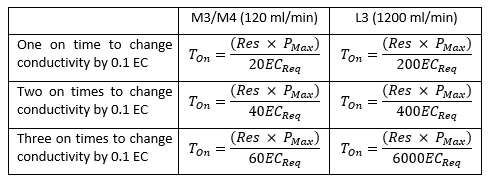1. Software guides
2. Pro Controller

# On Time Calculation

There are a couple of ways of calculating the On Time an initial guess at time, measuring liquid or via a formula. For all of these we start with the fact that one On Time should move our measurement by 0.1EC (1 CF, 50 ppm500 or 70 ppm700), 0.1 pH or 1 °C/°F. Any more than this and you risk overshooting.

### An Initial Guess at Time

This method will only work for conductivity or pH. Start with either a fresh tank or a tank that is far enough away from the ideal value that you have some room to play around then follow these steps;

• Take a guess at how much nutrient you think you would need to shift the measure and set the On Time for double that value
• Put the Controller in Control mode and let it carry out one On time
• Once the On time is done switch the controller back to Monitor mode so it doesn’t dose again
• Wait for the EC/Temp or pH value (whichever you are calculating) stabilise and make a note of that time
• Once it is stabilised see what increase the selected On Time gave and correct to make it 0.1EC (1 CF, 50 ppm500 or 70 ppm700), 0.1 pH or 1 °C/°F
• Eg. if an On Time of 30 seconds gave a change of 0.3 EC, change your On Time to 10 seconds as this should give 0.1 EC
• After applying the setting set the controller in Control Mode again and check that the level increases by only the desired amount
• Repeat until you have the system dialled in

### Measuring Liquid

This method will only work for conductivity or pH. Start with either a fresh tank or a tank that is far enough away from the ideal value that you have some room to play around, then follow the steps below. Note that if you are looking to calculate pH on time the tank should have nutrient in it to act as a buffer. To create a stable pH reading the minimum EC level should be 0.2 EC.

• Add nutrients to measuring jugs or similar so that you can see how much is being used
• Slowly add small quantities of pH adjuster or nutrients in the correct proportions (if nutrient A and B should be added in equal amounts be sure to do this whilst adding nutrients in this step)
• Wait for the nutrients to mix and continue adding and letting mix until the value (conductivity or pH) has increased by 0.1
• Add the same amount again and let mix to verify this is the correct amount
• Now use this ml value to calculate how long the pumps need to run to add that amount (remember if using 2 part nutrients you need to half the total ml value as each nutrient will have its own pump)
• For example an M3 or M4 doses 120 ml/minute, or 2 ml/second, so if it takes 140ml of nutrient A to increase the tanks EC by 0.1 you will need the pump running for 70 seconds (140ml / 2ml/s), so this would be your on time.

### By formula

The last option is to calculate the on time using a formula. Note this will only work for nutrient dosing.

Here use one of the following formula based on what pump type you have and how many does you want to make a 0.1 EC change;Where;

• Ton = On time
• Res = Resevoir/tank size (litres or gallons)
• PMax = Maximum pump amount (must be in ml/l if res size is in litres or ml/gal if res size is in gallons)
• ECReq = Required EC